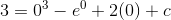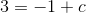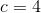# AP Calculus BC : Initial Conditions

## Example Questions

### Example Question #62 : Integrals

The temperature of an oven is increasing at a rate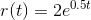degrees Fahrenheit per miniute for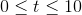minutes. The initial temperature of the oven is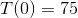degrees Fahrenheit.

What is the temperture of the oven at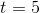? Round your answer to the nearest tenth.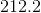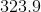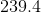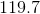Explanation:

Integrating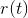over an interval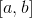will tell us the total accumulation, or change, in temperature over that interval. Therefore, we will need to evaluate the integral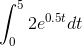to find the change in temperature that occurs during the first five minutes.

A substitution is useful in this case. Let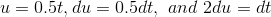. We should also express the limits of integration in terms of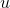. When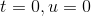, and when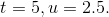Making these substitutions leads to the integral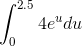.

To evaluate this, you must know the antiderivative of an exponential function.

In general,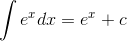.

Therefore,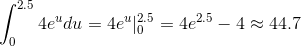This tells us that the temperature rose by approximately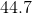degrees during the first five minutes. The last step is to add the initial temperature, which tells us that the temperature atminutes is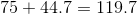degrees.

### Example Question #63 : Integrals

Find the equation for the velocity of a particle if the acceleration of the particle is given by: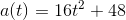and the velocity at time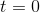of the particle is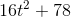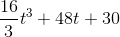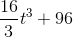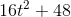Explanation:

In order to find the velocity function, we must integrate the accleration function: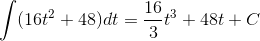We used the rule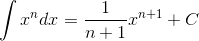to integrate.

Now, we use the initial condition for the velocity function to solve for C. We were told that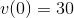so we plug in zero into the velocity function and solve for C: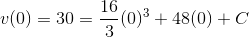C is therefore 30.

Finally, we write out the velocity function, with the integer replacing C: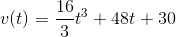### Example Question #64 : Integrals

Find the work done by gravity exerting an acceleration of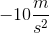for a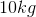block down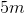from its original position with no initial velocity.

Remember that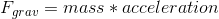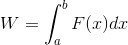, where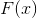is a force measured in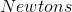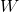is work measured in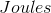, andandare initial and final positions respectively.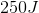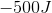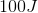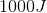Explanation:

The force of gravity is proportional to the mass of the object and acceleration of the object.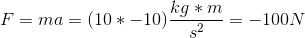Since the block fell down 5 meters, its final position isand initial position is.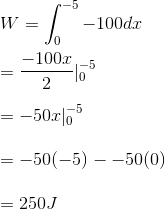### Example Question #65 : Integrals

Evaluate the following integral and find the specific function which satisfies the given initial conditions: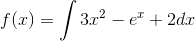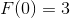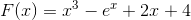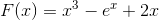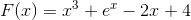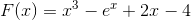Explanation:

Evaluate the following integral and find the specific function which satisfies the given initial conditions:To solve this problem,we need to evaluate the given integral, then solve for our constant of integration.

Let's begin by recalling the following integration rules: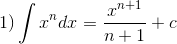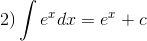Using these two, we can integrate f(x)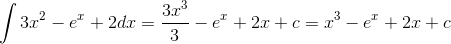SO, we get: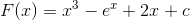We are almost there, but we need to find c. To do so, plug in our initial conditions and solve: